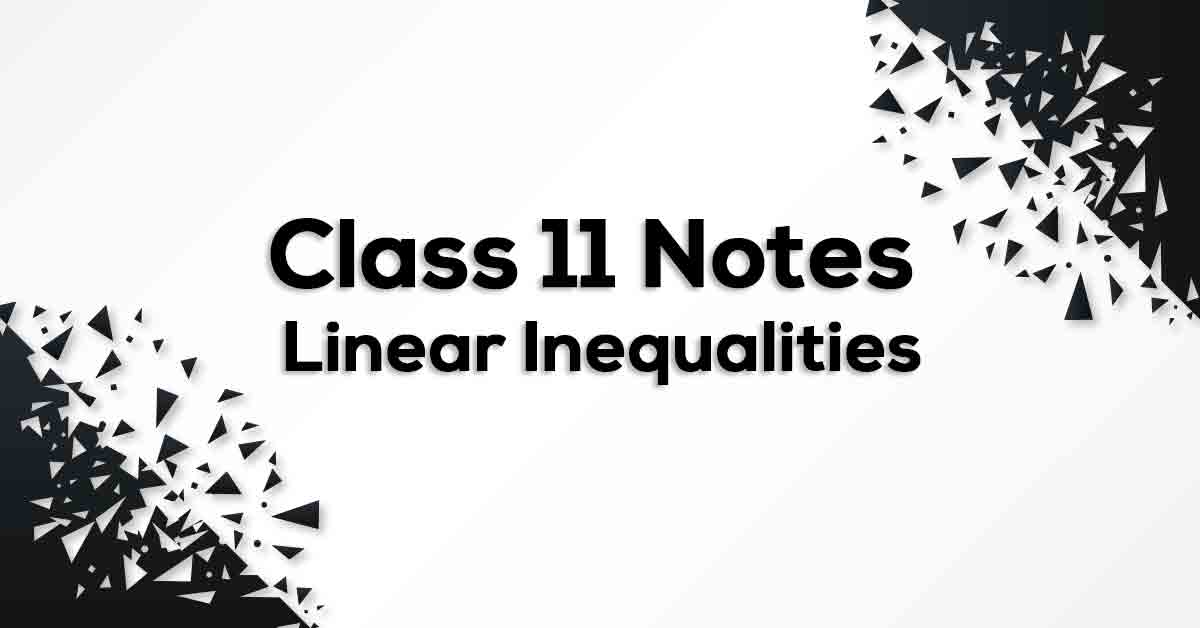Linear Inequalities Class 11 Notes | Vidyakul
×

# Linear Inequalities Class 11 Notes## Class 11 Math Chapter 6 Linear Inequalities Formulae - PDF Download

Linear Inequalities Class 11 Notes are cumulated in a systematic manner which gets rid of confusion among children regarding the course content since CBSE keeps on updating the course every year. The formulae list covers all formulae which provides the students a simple way to study of revise the chapter. Linear Inequalities Class 11 Notes would provide you with an effective preparation for your examinations.

### What do you understand by Linear Inequalities?

In mathematics a linear inequality is an inequality which involves a linear function. A linear inequality contains one of the symbols of inequality: < is less than. > is greater than. ≤ is less than or equal to. Linear inequalities in one variable: An inequality which involves a linear function in one variable E.g. x < 5; Linear inequalities in two variables: An inequality which involves a linear function in two variables. E.g. 3x + 2y < 5; Quadratic inequalities: An inequality which involves a quadratic function. E.g. x2 + 2x ≤ 5